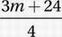# SAT Math Multiple Choice Question 694: Answer and Explanation

### Test Information

Question: 694

4. The average (arithmetic mean) of a set of 3 positive integers is m. If the number 24 is added to this set, what is the average (arithmetic mean) of the new set of numbers?

• A.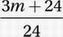• B.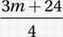• C. m + 8
• D.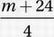Explanation:

B

Data Analysis (central tendency) EASY

Let's call the 3 positive integers a, b, and c. If the average of these numbers is m, then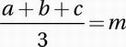Multiply by 3:

a + b + c = 3m

New average when 24 is included in the set: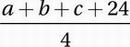Substitute a + b + c = 3m: